ISSAC 97 - Meijer G Function Representations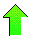Contents News View

 Strategy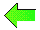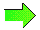Assume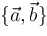and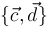are disjoint.  Suppose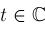and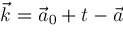,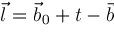,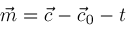,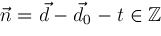.  We would try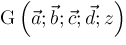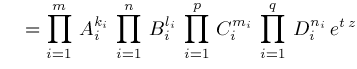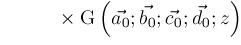but this will not always work because of restrictions on where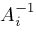,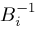,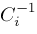, and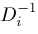are defined.

Given any vector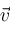, let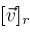be the subvector of elements ofwhich are congruent to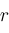mod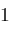.  Given any permutation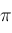of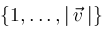let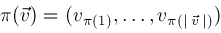Let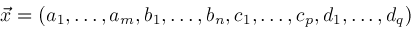Letbe a permutation which sorts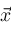into nondescending order.  Let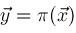.  Then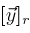is nondescending for every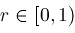Assume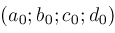is a suitable origin such thatand,,,.  Let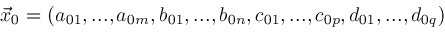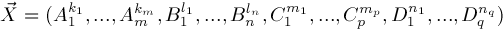Let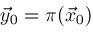and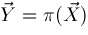.  Assume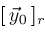is nondescending for everyFor any given, plot the elements ofand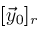as a function of position.  Call the resulting monotonic polygonal curves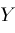and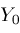.  For example, we might get this picture: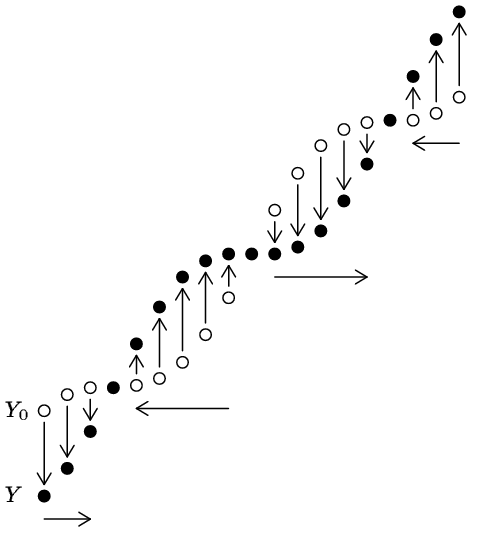To avoidandhaving elements in common as we apply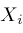operators to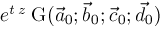we may proceed left to right wherelies belowand right to left wherelies above or onLet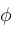be a permutation ofthat in every plot ofandfor everyselects the elements offrom left to right wherelies belowand selects the elements offrom right to left wherelies above or on.  Then we should applyoperators to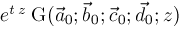in the order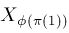,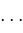,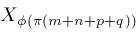.  That is,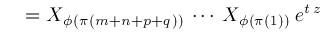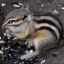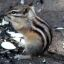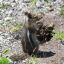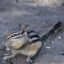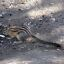©2004-2021 Planet Quantum Kelly Roach